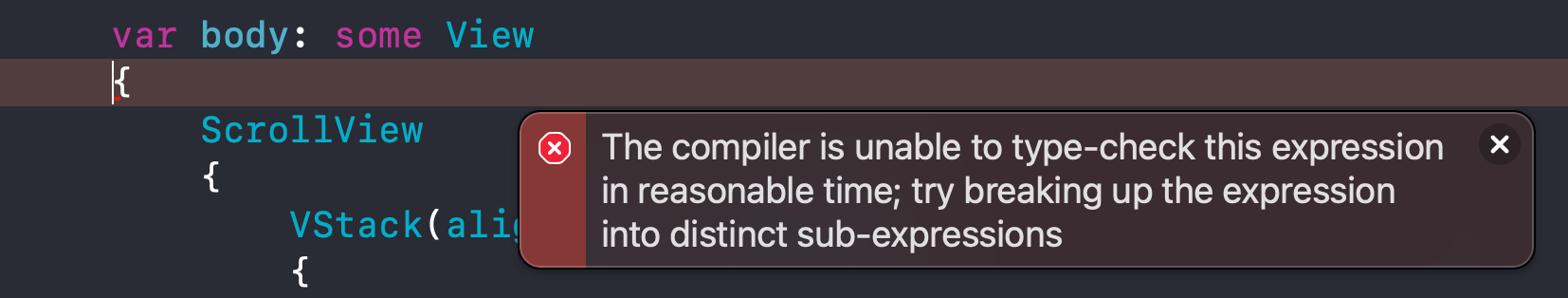Once in a while, you might come across this error:

The compiler is unable to type-check this expression in reasonable time; try breaking up the expression into distinct sub-expressionsThe compiler is unable to type-check this expression in reasonable time; try breaking up the expression into distinct sub-expressions.

There might be several reasons that cause this error. I can show you one way to fix it.

## Cause of the problem

The cause of this can vary, but it boils down to quite the same thing.

That is the combination of expressions, e.g., `=`, `+`, `try`, `await`, is too complex for Swift compiler.

You might be surprised that the code that causes the error isn't that complex to the human eye.

Here is an example of code that can cause the error.

``Text(String(index + 1) + " - " + recipe.directions[index])``

You can easily support sarunw.com by checking out this sponsor.

Sponsor sarunw.com and reach thousands of iOS developers.

## How to fix "the compiler is unable to type-check this expression in reasonable time" error

Since the cause can vary and the problematic code is hard to notice. I can only give you a rule of thumb to solve this problem.

First, we need to locate the code that causes the error.

Here is how you find one.

1. Find chunk of code that contain a lot of expression. If you don't know where to start, a code containing many plus operations (`+`) might be a good start.
2. Comment out the suspicious chunk of code and rebuild to see whether the error is gone.
3. Repeat step two until you find a code that causes the problem.

Once you find the problematic code, you have to make it simpler.

There are many ways to do it. I can give you two ways to tackle this.

### Break a long expression into smaller expressions

In this example, we break one expression into three smaller ones.

The total number of expressions is the same (three plus operations), but each expression contains less (one plus operation for each expression).

``// Fromvar longExpression = "a" + "b" + "c" + "d"    // Tovar temp1 = "a" + "b"var temp2 = "c" + "d"var smallerExpression = temp1 + temp2``

### Reduce the amount of expression

In this example, we reduce the number of plus operations (`+`) from three to one.

``// Fromvar longExpression = "a" + "b" + "c" + "d"    // Tovar reduceExpression = "ab" + "cd"``

Here is a real-world example. The code `Text(String(index + 1) + " - " + recipe.directions[index])` cause the "the compiler is unable to type-check this expression in reasonable time" error.

I can fix it by reducing the amount of expression by converting it to `Text("\(index + 1) - \(recipe.directions[index])")`.

``// FromText(String(index + 1) + " - " + recipe.directions[index])// ToText("\(index + 1) - \(recipe.directions[index])")``

We reduce the number of plus operations from two to zero.

1. The `+` operator is heavily overloaded, it contain around 60 different functions. ↩︎

Every Friday, you'll get a quick recap of all articles and tips posted on this site. No strings attached. Unsubscribe anytime.

If you enjoy my writing, please check out my Patreon https://www.patreon.com/sarunw and become my supporter. Sharing the article is also greatly appreciated.

Previous
SwiftUI Dynamic List View

Learn how to create a list from a collection of data.

Next
SwiftUI Button Style Examples

In this article, I will show you all 5 button styles you can use with SwiftUI Button in iOS.

← Home